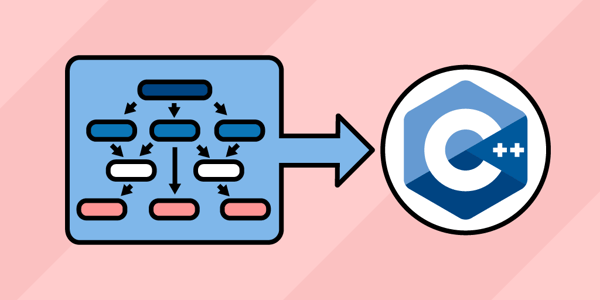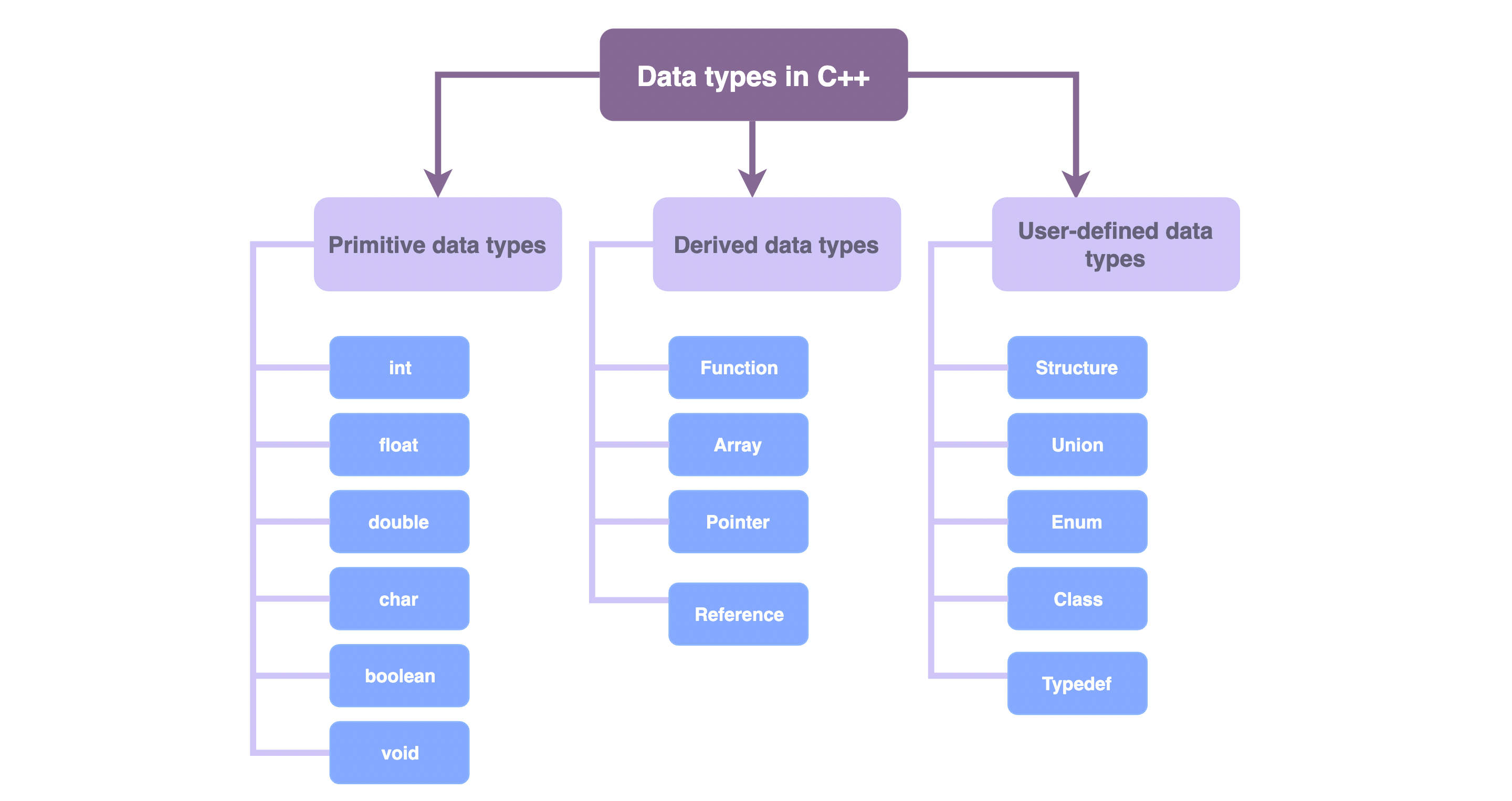Home/Blog/Tutorials & Guides/How to pass an array to a function in C++

# How to pass an array to a function in C++

Dec 09, 2021 - 8 min readErica VartanianArrays are useful data types for data structures in C++ programming and beyond. In simple terms, arrays allow you to store data as an ordered list. You can use arrays to easily sort or calculate information about a collection of data using a single array name.

Today, we’ll discuss how to pass arrays to functions in C++. Along the way, we’ll cover why we use C++ arrays, as well as how to declare and initialize them. We’ll then pass them to functions and discuss important distinctions about arrays in C++. This article includes tutorials for both one-dimensional and two-dimensional arrays.

We’ll cover:

## Arrays and data structures in C++

Arrays are part of a bigger concept in computer science: data structures. We use data structures to organize and store data in order to efficiently perform operations on that data. Data structures are composed of smaller components called data types. Arrays are a data type frequently used in data structures.As illustrated in the diagram, C++ has the following data types:

• Primitive (or fundamental) data types
• Derived data types
• User-defined data types

Arrays are derived data types. Derived data types are composed of primitive or built-in data types. Derived data types have some of the abilities that primitive data types lack. For instance, primitive data types can’t store more than one value at a time, while arrays can.

Arrays allow you to store and access multiple values of the same data type under a single name. Arrays are useful when you’re dealing with a large amount of data. For instance, given data on 100 students and their grades, it would be tedious to declare 100 variables to store each student’s grade. Instead, we’d use an array to store all the data under a single name.

Basic terms associated with arrays are elements of the array, index of the array, and size of the array.

• Element: Refers to values stored in an array (at neighboring memory locations)
• Index: Refers to an array element’s position, starting from 0 and increasing incrementally by one for each additional element
• Size: Refers to the total number of elements stored in an array

Here’s an illustration of an array with six elements. The array size is six according to the number of elements. Each of the six elements are stored at their respective indexes (e.g. the first element of the array, 10, is stored in index zero).A two-dimensional array (or 2-d array) is an array of arrays. Two-dimensional arrays represent a matrix. They are the most commonly used multidimensional arrays. We can access the elements in a two-dimensional array by specifying their row and column index, which start at zero and increase incrementally with each added element.

This matrix illustration represents an array of size nine. Each of the nine elements are stored at a respective row and column index.A pointer is another derived data type that’s relevant to today’s discussion. We’ll discuss more about pointers toward the end of this article.

## Array declaration in C++

### How to declare a one-dimensional array

The general syntax for declaring a one-dimensional array involves specifying the data type, name of the array, and size of the array:

DataType ArrayName [ArraySize];

In this code, we declare a one-dimensional array Roll_Number that can store five integer values. The compiler reserves space for five elements of int data type consecutively in memory.

#include <iostream>using namespace std;int main() {  int Roll_Number;  }

### How to declare a two-dimensional array

The general syntax for declaring a two-dimensional array involves specifying the data type, array name, row index, and column index:

DataType ArrayName [RowSize] [ColumnSize];

In this code, we declare a two-dimensional array Student that can hold 10 arrays of Student. Each Student array can store five integer values. The compiler reserves space for 50 elements (10*5) of the int data type consecutively in memory.

#include <iostream>using namespace std;int main() {  int Student;  }

## Initializing an array in C++

### How to initialize a one-dimensional array

The general syntax for initializing a one-dimensional array is:

ArrayName [ArrayIndex] = value;

In this code, we initialize an array named Roll_Number that stores: 100 at index 0, 101 at index 1, 102 at index 2, and so on.

#include <iostream>using namespace std;int main() {  int Roll_Number;  Roll_Number = 100;  Roll_Number = 101;  Roll_Number = 102;  Roll_Number = 103;  Roll_Number = 104;}

### How to initialize a two-dimensional array

The general syntax for initializing a two-dimensional array is:

ArrayName [RowIndex] [ColumnIndex] = Value;

This code initializes a two-dimensional array that stores: 100 at row index 0 and column index 0, 134 at row index 0 and column index 1, and so on.

#include <iostream>using namespace std;int main() {  int Student;  Student = 100;  Student = 134;  Student = 34;  Student = 189;  }

## Declaring and initializing arrays in one step

### How to declare and initialize one-dimensional arrays

The general syntax to initialize and declare a one-dimensional array is:

DataType ArrayName [ ] = {value1, value 2,…, valueN}

In this example, we initialize the Roll_Number array in the declaration step.

#include <iostream>using namespace std;int main() {  int Roll_Number[ ] = { 100, 101, 102, 103, 104 };}

### How to declare and initialize two-dimensional arrays

The general syntax to initialize and declare a two-dimensional array is:

DataType ArrayName [] [] = {{value1,…,valueN},…{value1,…,valueN}}

In this example, we initialize the Student[] array in the declaration step. Specifying the first dimension is optional when initializing two-dimensional arrays. The compiler will infer the number of rows from your statement. That’s why the code in this example is written with Student[] instead of Student.

#include <iostream>using namespace std;int main() {  int Student[] = {{100, 134, 234}, {34, 189, 221}, {109, 139, 56}};}

## Passing arrays to functions in C++

Now that we’ve covered the basics, let’s start passing arrays to C++ functions. We can use array names as parameters during function declaration.

To pass arrays to a function, we specify the array type, followed by the name of the array and square brackets in the function parameters. The square brackets tell the compiler that the first function parameter is an array, otherwise, a variable will be assumed.

### Passing one-dimensional arrays to functions

The general syntax for passing an array to a function in C++ is:

FunctionName (ArrayName);

In this example, our program will traverse the array elements. We’ll modify the value of any element less than 50 to a value of -1.

#include <iostream>using namespace std;// print_array function will print the values of an arrayvoid print_array(int number[], int size) {  for (int i = 0; i < size; i++) {    cout << number[i] << " ";  }  cout << endl;}// modify_array functionvoid modify_array(int number[], int size) {  // Traverse array  for (int i = 0; i < size; i++) {    // If value less tha 50 set it to -1    if (number[i] < 50)      number[i] = -1;  }  cout << "Values of array inside the function:" << endl;  // Call print_array function   print_array(number,size);}// main functionint main() {  // Initialize size of an array  int size = 8;  // Initialize values of array  int number[size] = {67, 89, 56, 43, 29, 15, 90, 67};  cout << "Values of array before function call:" << endl;  // Call print_array function  print_array(number,size);  // Call modify_array function  modify_array(number, size);  cout << "Values of array after function call:" << endl;  // Call print_array function  print_array(number,size);}

### Passing two-dimensional arrays to functions

In this example, we’ll pass the two-dimensional array int n[] to a function we define as display() and print the array’s elements. For the function call, we only pass the array’s name as the function argument display(num).

#include <iostream>using namespace std;// define function // pass 2-d array as a parametervoid display(int n[]) {    cout << "Displaying Values: " << endl;    for (int i = 0; i < 3; ++i) {        for (int j = 0; j < 2; ++j) {            cout << "num[" << i << "][" << j << "]: " << n[i][j] << endl;        }    }}int main() {    // initialize 2-d array    int num = {        {3, 4},        {9, 5},        {7, 1}    };    // call function    // pass 2-d array as argument    display(num);    return 0;}

## Arrays: Pointers and passing by reference

You may have noticed that we didn’t specify the size of the array when passing them to functions. The reason for this has to do with the fact that, by default, arrays are passed by reference to arguments. This is different from the case for variables, which are passed by value. When we use an array name as a function parameter, we’re actually passing the original array to the function. Let’s expand on exactly what that means.

Arrays are passed by reference for practical reasons. For instance, imagine we had a large array with a size of 10,000. It would be taxing to pass the entire array and its 10,000 elements. In fact, we actually can’t pass an entire array to a function in C++. Under the hood, what we’ve been doing all along is passing a pointer, which contains a memory address to the first element of the array.

We mentioned pointers earlier as another derived data type. Pointers hold memory addresses of other data types. When we use a pointer type as a function parameter instead of a data value, we’re giving the function a memory address directly to the specific piece of data. For objects that are passed by reference to a function, any change to the formal function parameter affects the actual parameter in the calling environment. This is because the formal parameter receives a reference (or pointer) to the actual data.

In C++, you technically get a pointer with every array declaration. Arrays and pointers are not equivalent, but their arithmetic is defined such that a pointer can serve to access or simulate an array. The name of the array acts as a pointer to the memory address of the array’s first element. When an array appears in an expression, the compiler generates a pointer to the address of the first element of the array.

## Wrapping up and next steps

You now know the basics of using arrays in C++. Arrays are data types that we can use to store a collection of data under a single name. We’ve covered why and how we work with one-dimensional and two-dimensional arrays. We also discussed the role of pointers when passing arrays to functions.

To continue learning, check out Educative’s interactive learning path: C++ for Programmers. This program covers topics such as arrays, loops, and templates.

Happy learning!